Mathematical and Physical Journal
for High Schools
Issued by the MATFUND Foundation
 Already signed up? New to KöMaL?

#Problem A. 547. (November 2011)

A. 547. A tetrahedron OA1A2A3 is given. For i=1,2,3 let Bi be a point in the interior of the edge OAi and let Ci be a point on the ray OAi, beyond Ai. Suppose that the polyhedron bounded by the six planes OAi+1Ai+2 and BiAi+1Ai+2 (i=1,2,3) circumscribes a sphere, and the polyhedron bounded by the planes BiAi+1Ai+2 and CiAi+1Ai+2 also circumscribes a sphere. Prove that the polyhedron bounded by the planes OAi+1Ai+2 and CiAi+1Ai+2 also circumscribes a sphere.

(5 pont)

Deadline expired on December 12, 2011.

Solution (outline). We will use oriented lines, planes and angles. For arbitrary, non-collinear points X,Y,Z, the orinetation of the line XY the same as the direction of the vector, and the vectorpoints into the positive side of the plane XYZ,

Lemma. Letbe an oriented line which is contained by the oriented planes,and. Letbe the angle by which the planecan be rotated toaround(i=1,2; these angles are considered modulo 360o). Suppose that some point X has signed distances d,r,-r from the planes,,, respectively. Then we haveProof. Denote bythe unit normal vector of the planewhich points to the positive side of the plane and letbe the direction vector of the lineLetbe a vector from a point ofto X. Then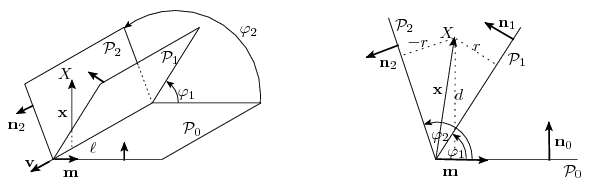Let.ThenTaking the dot-product with,sin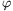2.r-sin1.(-r)=sin (2-1).dFrom this we getThe lemma is proved.

Let X and rX be the center and radius of the sphere, respectively, which is circumcribed by the planes OAi+1Ai+2 and BiAi+1Ai+2. Let dX be the signed distance between X and the plane A1A2A3 síktól dX. The signed distances between X and the planes BiAi+1Ai+2 are all rX, and the signed distances between X and the planes OAi+1Ai+2 are all -rX.

Analogously, let Y and rY be the center and radius of the sphere which is circumscribed by the planes BiAi+1Ai+2 and CiAi+1Ai+2. let dY by the signed distance between Y and the plane A1A2A3. The signed distances between Y and the planes CiAi+1Ai+2 are all rY, and the signed distances between X and the planes BiAi+1Ai+2 are all -rY.

For every i=1,2,3 take the external angle bisector of the planes Ai+1Ai+2O and Ai+1Ai+2Ci, and let Z be the intersection point of the three angle bisector planes --- Z will be center of the desired sphere. (Now assume that none of the angle bisectors coincides with the plane A1A2A3; then the point Z is unique and does not lie on A1A2A3.)

Let dZ and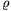be the signed distance between Z and A1A2A3 and A1A2C3, respectively. Since Z lies on the external angle bisector between A1A2C3 and A1A2O, the signed distance between Z and A1A2O is -.

Let=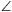(A1A2A3,A1A2O),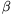=(A1A2A3,A1A2B3) and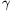=(A1A2A3,A1A2C3), where the angles are measured around the oriented line A1A2.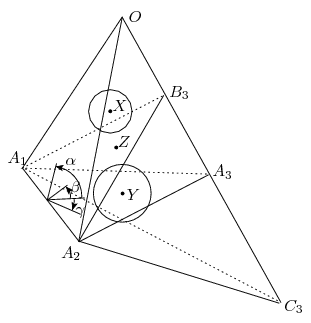Applying the Lemma for the line A1A2, the planes A1A2A3, A1A2B3, A1A2O and the point X we get(1)

Similarly, applying the Lemma for the line A1A2, the planes A1A2A3, A1A2C3, A1A2B3 and the point Y we get(2)

Finally, Similarly, applying the Lemma for the line A1A2, the planes A1A2A3, A1A2C3, A1A2O and the point Y we obtainAs can be seen, due to dZ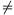0 the distances dX,rX,dY,rY,dZ determine. If we cyclicly shift the indices 1,2,3, we obtain the same value. Hence, the point Z has the same distance from all planes OAi+1Ai2 and CiAi+1Ai2.

In the particular case when some planes CiAi+1Ai+2 and OAi+1Ai+2 are symmetric over A1A2A3, the points C1,C2,C3 can be moved in such a way that the three spheres exist; then the original configuration can be considered as a limit case.

### Statistics:

 2 students sent a solution. 0 point: 2 students.

Problems in Mathematics of KöMaL, November 2011